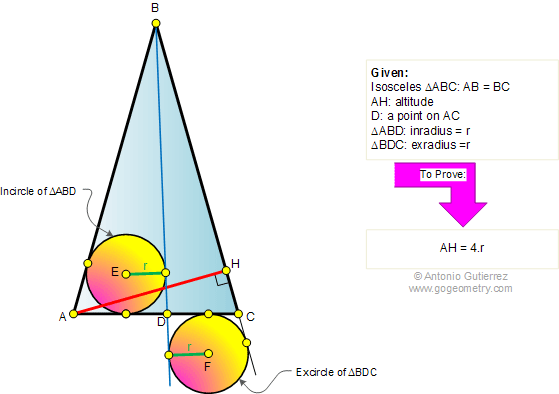v Online Geometry Tutoring Problem 325. Isosceles triangle, Altitude, Cevian, Incircle, Excircle. Math teacher Master Degree. College, SAT Prep. Elearning, Online math tutor, LMS
 Problem 325. Isosceles triangle, Altitude, Cevian, Incircle, Excircle. Level: High School, SAT Prep, College geometry. The figure shows an isosceles triangle ABC with AB = BC. AH is an altitude and D is a point on AC. The incircle of triangle ABD and the excircle of triangle BDC, relative to CD, are equal circles with radius r, Prove that AH = 4.r .Recent Additions